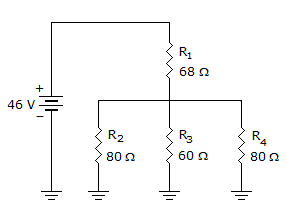# Electronics - Series-Parallel Circuits - Discussion

### Discussion :: Series-Parallel Circuits - General Questions (Q.No.15)

15.How much voltage is dropped across R3 in the given circuit?

 [A]. 46 V [B]. 34 V [C]. 23 V [D]. 12 V

Explanation:

No answer description available for this question.

 Harshitha said: (May 4, 2011) Parallel resistance along R2,R3,R4=24 Total resistance= R1+(R2||R3||R4) =92 Total current= (v/i)= 46/92 =0.5A Voltage drop across R3= 24*0.5= 12v

 Salini said: (Sep 30, 2015) I am not getting 24 in parallel resistor value.

 Pinkie said: (Oct 20, 2015) Meaning of ||?

 Prathmesh said: (Dec 2, 2015) || means parallel.

 Balasabarish said: (Dec 29, 2015) Parallel resistance along R2, R3, R4 = 24. Total resistance = R1+ (R2||R3||R4) = 92. Total current = (v/i) = 46/92 = 0.5 A. Voltage drop across R1 = Total current*R1 = 34. Voltage drop across R3 = Source Voltage - Voltage drop across R1. = 46-34 = 12 V.

 Hamsanandhini said: (May 30, 2016) It is very easy. Thanks for the explanation.

 Raja said: (Oct 17, 2016) @Balasabarish. Why did you use R1*.5. Should you use R3*.5 rite?

 Rohit said: (Nov 16, 2018) In question, it asked voltage drop at R3 terminal and 24 is the parallel resistance of the circuit.

 Kumar said: (Aug 1, 2019) Parallel resistance along R2, R3, R4 = 24. Total resistance= R1+(R2||R3||R4) = 92, Total current= (v/i)= 46/92 =0.5A, Voltage drop across R3= 24 * 0.5 = 12.

 Varun said: (Jan 28, 2021) What is parallel resistance along R2, R3, R4?